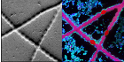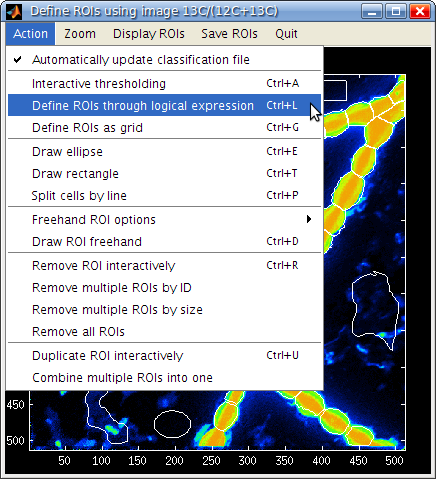#nanosims-wiki

### Sidebar

nanosims:lans_extras:rois_logical_expression

## Definition of ROIs through a logical expression

2013-01-05:

I want to define ROIs (regions of interest) as contiguous groups of pixels that satisfy a certain condition. For example, I want to use the 12C14N image as a template for ROI definition, and define ROIs by selecting all pixels where the accumulated 12C14N- ion counts are larger than 3000.

#### Possible solution

LANS allows you to execute a more general version of this idea.

1. Select Define ROIs through logical expression in the Action menu of the Interactive ROIs definition tool (see image).2. In the input dialog box that pops up, enter the logical expression. Note that this must be a valid conditional statement written in Matlab syntax.

#### Examples

1. First we assume that the ROI template image consists of a single image (e.g., ion counts image, or a ratio image). Using expression `r>0.5` will select all contiguous regions for which the pixel value in the image is larger than 0.5. Similarly, expression `r>0.5 & r<1` will select regions for which the pixel values are between 0.5 and 1. Use `|` if you want to specify a condition with logical or (e.g., `r<0.1 | r>0.5`).
2. Now we assume that the ROI template is a composite RGB image derived from a combination of two or three ion counts or ratio images. In this case the logical expression can be formulated using all three channels of the image (r=red, g=green, b=blue). For example, possible logical expressions could be: `r>0.2 & g<0.5 & b>2`, or `(r>0.5 | g<0.2) & b>2`.

#### Few notes

1. ROIs defined in this way will always have more than 4 pixels. This is to avoid getting too many small ROIs if a grainy/noisy image is used as the ROI definition template.
2. To minimize the effects of pixel-to-pixel noise on the shape and contiguity of the ROIs defined in this way, it is recommended to use a smoothed template image. This is done by entering a kernel size of for example 3 or 5 in the Smoothing kernel field before selecting Interactive ROIs definition tool from the Action menu of LANS.